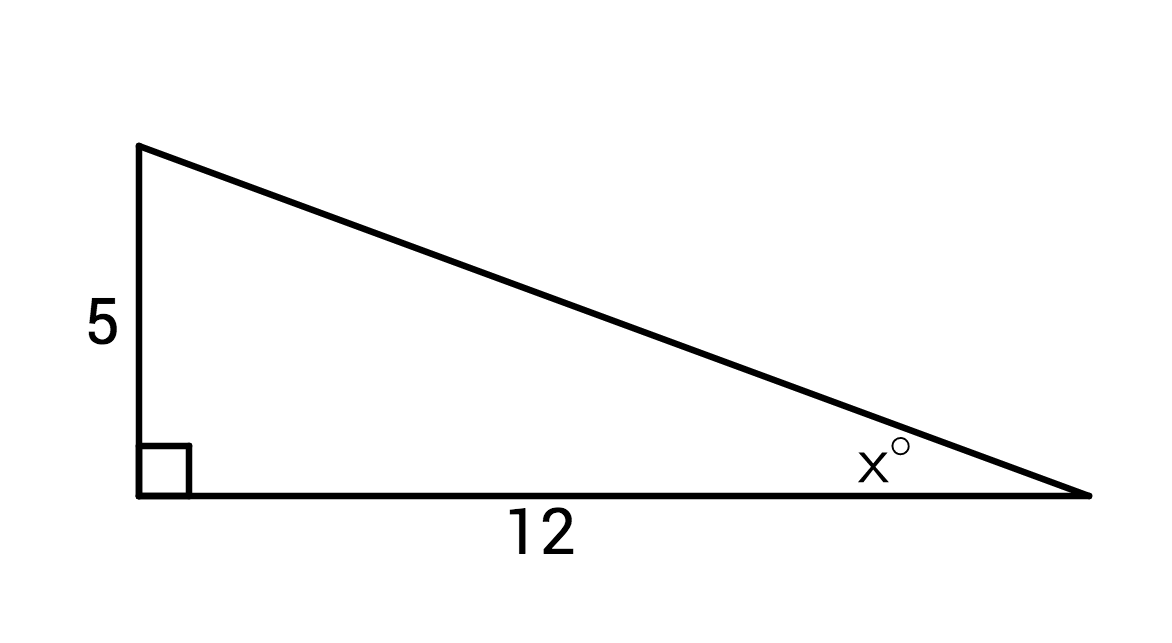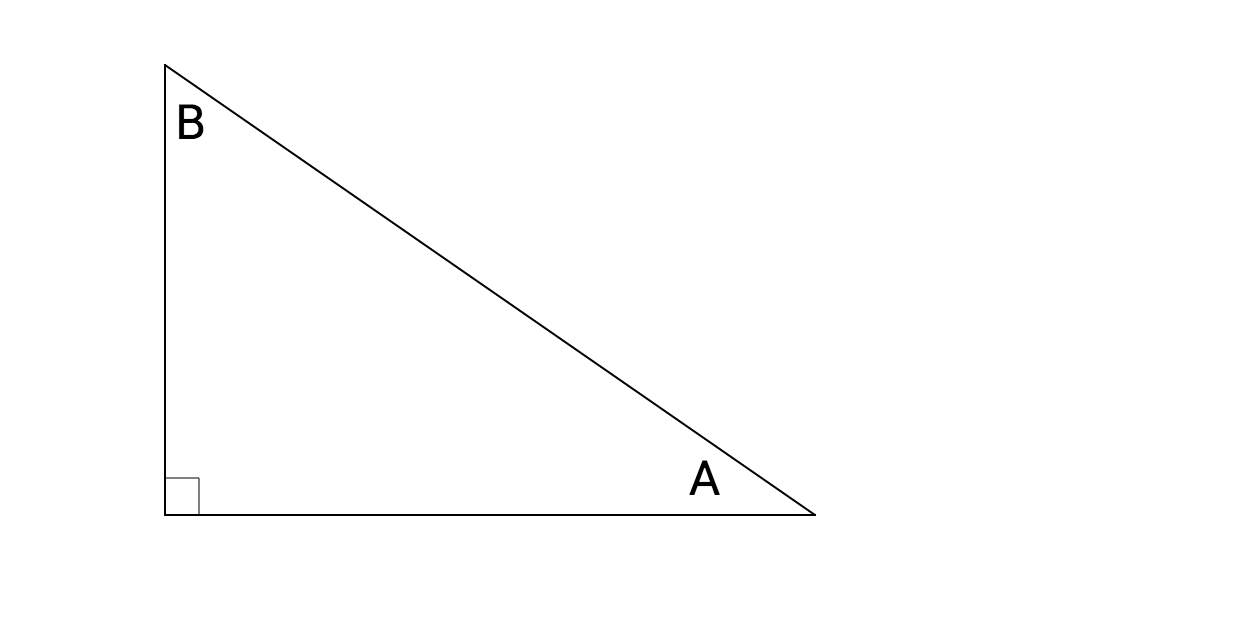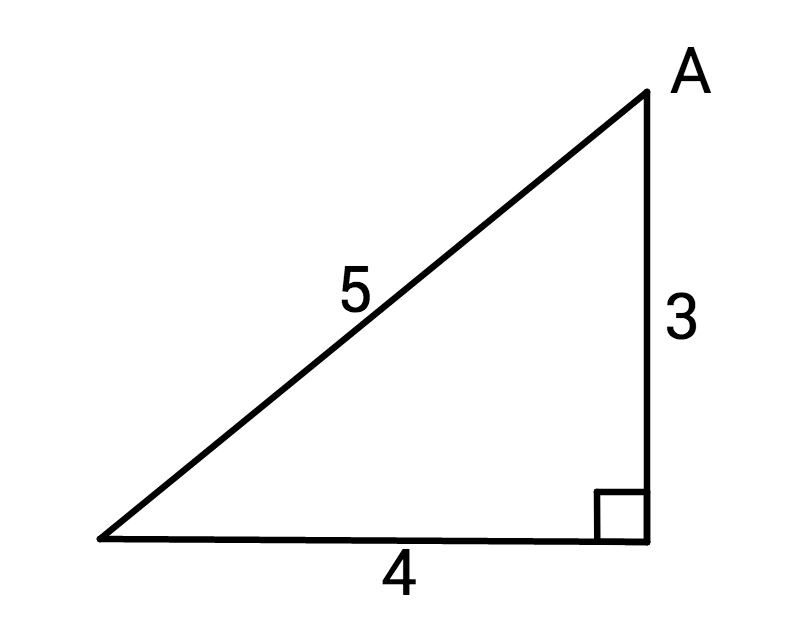Geometry

Trigonometry WarmupIf $\tan(x) = \frac {5}{12}$, what is the value of $\sin(x)$?In the triangle shown, $\sin \angle$ A = ?

If $\sin(x) = 0.6,$ what is $\cos(90^\circ - x)?$

Which characteristic best describes the graph of $y = \sin x$?Which equation could be used to determine the measure of angle $A ?$

×